# Selina Concise Mathematics Class 10 ICSE Solutions Chapter 1 Value Added Tax Ex 1C

## Selina Concise Mathematics Class 10 ICSE Solutions Chapter 1 Value Added Tax Ex 1C

These Solutions are part of Selina Concise Mathematics Class 10 ICSE Solutions. Here we have given Selina Concise Mathematics Class 10 ICSE Solutions Chapter 1 Value Added Tax Ex 1C.

Other Exercises

[In this exercise, all the prices are excluding tax/VAT unless specified].
Question 1.
Madan purchases a compact computer system for ₹ 47,700 which includes 10% rebate on the marked price and then 6% Sales Tax on the remaining price. Find the marked price of the computer.
Solution:
Sales price of the computer = ₹ 47700
Rate of Sales Tax = 6%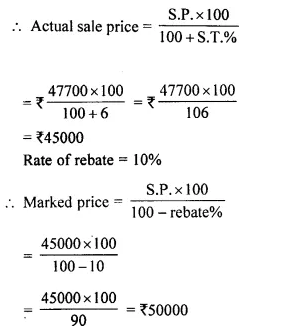Hence marked price = ₹ 50,000

Question 2.
An article is marked at ₹ 500. The wholesaler sells it to a retailer at 20% discount and charges sales-tax on the remaining price at 12.5%. The retailer, in turn, sells the article to a customer at its marked price and charges sales-tax at the same rate. Calculate :
(i) the price paid by the customer.
(ii) the amount of VAT paid by the retailer.
Solution:
Marked price of an article = ₹ 500
Rate of discount = 20%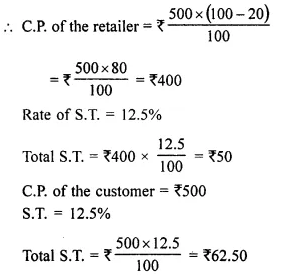(i) Price paid by-the customer = ₹ 500 + ₹ 62.50 = ₹ 562.50
(ii) VAt paid by the retailer = ₹ 62.50 – ₹ 50.00 = ₹ 12.50

Question 3.
An article is marked at ₹ 4,500 and the rate of sales-tax on it is 6%. A trader buys this article at some discount and sells it to a customer at the marked price. If the trader pays ₹ 81 as VAT; find :
(i) how much per cent discount does the trader get ?
(ii) the total money paid by the trader, including tax, to buy the article.
Solution:
Marked price = ₹ 4500
Rate of S.T. = 6%
VAT paid by the trader = ₹ 81
C.P. for the customer = ₹ 4500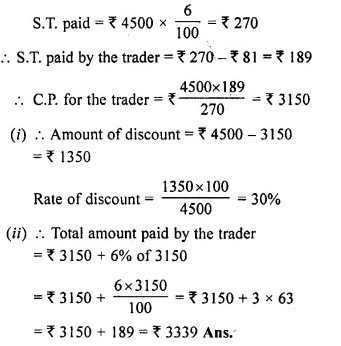Question 4.
A retailer sells an article for ₹ 5,350 including 7% Sales Tax on the listed price. If he had bought it at a discount and has made a profit of 25% on the whole, And the rate of discount he gets.
Solution:
S.P. of an article of the retailer (including S.T.) = ₹ 5350
Rate of S.T. = 7%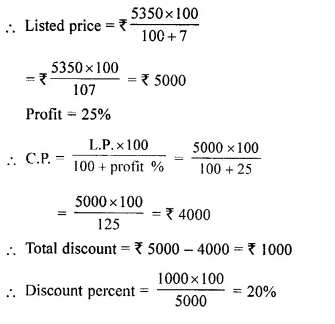Question 5.
A shopkeeper buys a camera at a discount of 20% from the wholesaler, the printed price of the camera being ₹ 1600 and the rate of sales tax is 6%. The shopkeeper sells it to the buyer at the printed price and charges tax at the same rate. Find :
(i) The price at which the camera can be bought from the shopkeeper.
(ii) The VAT (Value Added Tax) paid by the shopkeeper. (2008)
Solution:
Printed price (M.P) = ₹ 1600
Rate of discount = 20%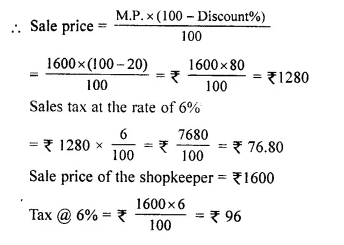(i) The buyers bought the camera = ₹ 1600 + ₹ 96 = ₹ 1696
(ii) VAT paid by the shopkeeper = ₹ 96 – ₹ 76.80 = ₹ 19.20

Question 6.
Tarun bought an article for ₹ 8000 and spent ₹ 1000 for transportation. He marked the article at ₹ 11,700 and sold it to a customer. If the customer had to pay 10% sales tax, find:
(i) The customer’s price
(ii) Tarun’s profit percent.
Solution:
Tarun’s cost price = ? 8000
Transportation charges = ₹ 1000
Tarun’s total cost = (₹ 8000 + ₹ 1000) = ₹ 9000
Tarun’s selling price = ₹ 11,700
Rate of sales tax = 10%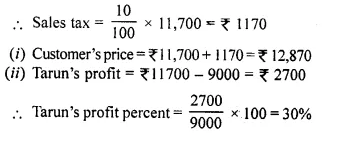Question 7.
A shopkeeper sells an article at the listed price of ₹ 1,500 and the rate of VAT is 12% at each stage of sale. If the shopkeeper pays a VAT of ₹ 36 to the Government, what was the price, inclusive of Tax, at which the shopkeeper purchased the articles from the wholesaler?
Solution:
Tax charged by shopkeeper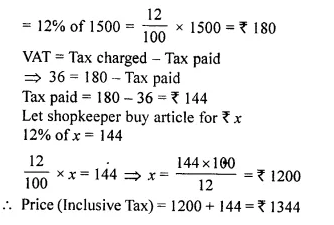Question 8.
A shopkeeper bought a washing machine at a discount of 20% from a wholesaler, the printed price of the washing machine being ₹ 18,000. The shopkeeper sells it to a consumer at a discount of 10% on the printed price. If the rate of sales tax under is 8%, find:
(i) the VAT paid by the shopkeeper.
(ii) the total amount that the consumer pays for the washing machine. (2014)
Solution:
(i) S.P. of washing machine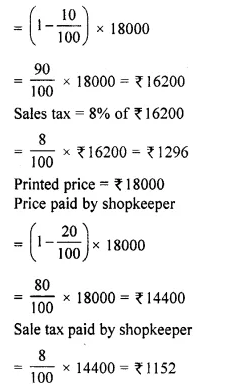VAT paid by shopkeeper = Tax charged – Tax paid = ₹ 1296 – ₹ 1152 = ₹144
(ii) Price paid by customer = ₹ 16200 + ₹ 1296 = ₹ 17496

Question 9.
Mohit, a dealer in electronic goods, buys a high class TV set for ₹ 61,200. He sells this TV set to Geeta, Geeta to Rohan and Rohan sells it to Manoj. If the profit at each stage is ₹ 2,000 and the rate of VAT at each stage is 12.5%, find :
(i) total amount of tax (under VAT) paid to the Government.
(ii) Money paid by Manoj to buy the TV set.
Solution:
For Mohit,
C.P. of electronic goods = ₹ 61200
Amount of profit in each case = ₹ 2000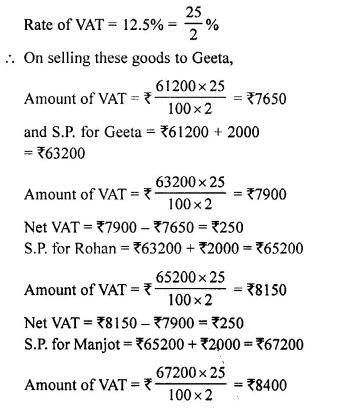Net VAT = ₹ 8400 – ₹ 8150 = ₹ 250
(i) Total amount of VAT paid to govt. = ₹ 7650 + ₹ 250 + ₹ 250 + ₹ 250 = ₹ 8400
(ii) Money paid by Manoj to buy the T.V. set = ₹ 67200 + ₹ 8400 = ₹ 75600

Question 10.
A shopkeeper buys an article at a discount of 30% of the list price which is ₹ 48,000. In turn, the shopkeeper sells the article at 10% discount. If the rate of VAT is 10%, find the VAT to be paid by the shopkeeper.
Solution:
Market value (M.P.) of an article = ₹ 48000
Rate of discount = 30%
Amount of discount = ₹ 48000 x $$\frac { 30 }{ 100 }$$ = ₹ 14400
(i) Cost price of shopkeeper = ₹ 48000 – ₹ 14400 = ₹ 33600
(ii) C.P. for shopkeeper = ₹ 33600
Rate of VAT = 10%
Amount of VAT paid by shopkeeper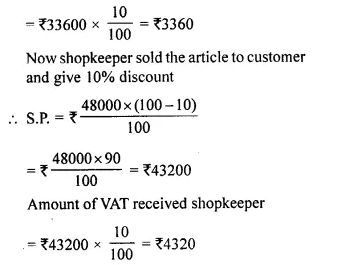Net VAT paid by the shopkeeper = VAT recovered from customer – VAT paid to dealer
= ₹ 4320 – ₹ 3360 = ₹ 960

Question 11.
A company sells an article to a dealer for ₹ 40,500 including VAT (sales-tax). The dealer sells it to some other dealer for ₹ 42,500 plus tax. The second dealer sells it to a customer at a profit of ₹ 3,000. If the rate of sales-tax under VAT is 8%, find :
(i) the cost of the article (excluding tax) to the first dealer.
(ii) the total tax (under VAT) received by the Government.
(iii) the amount that a customer pays for the article.
Solution:
For a company,
S.P. of an article including VAT = ₹ 40500
Rate of VAT = 8%
(i) Amount of article excluding VAT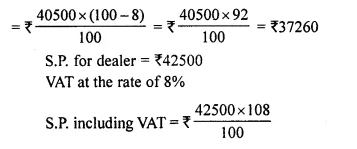= ₹ 425 x 108 = ₹ 45900
Total tax (VAT) = ₹ 45900 – ₹ 42500 = ₹ 3000
S.P. for the second dealer = ₹ 42500 + ₹ 3000 = ₹ 45500
(ii) VAT = ₹ $$\frac { 45500 x 8 }{ 100 }$$ = ₹ 3640
(iii) Total amount paid by customer = ₹ 45500 + ₹ 3640 = ₹ 49140

Question 12.
A wholesaler buys a TV from the manufacturer for ₹ 25,000. He marks the price of the TV 20% above his cost price and sells it to a retailer at 10% discount on the marked price. If the rate of VAT is 8%, find the :
(i) marked price.
(ii) retailer’s cost price inclusive of tax.
(iii) VAT paid by the wholesaler. (2015)
Solution:
S.P. for the manufactures = ₹ 25000
or C.P. for a whole seller a T.V. = ₹ 25000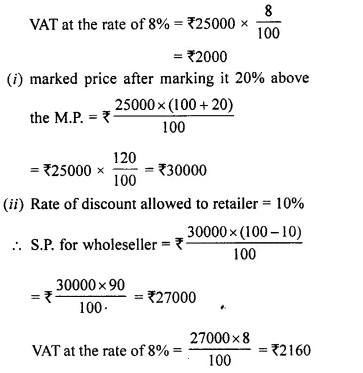C.P. for the retailer = ₹ 27000 + ₹ 2160 = ₹ 29160
(iii) VAT paid by the whole seller = ₹ 2160 – ₹ 2000 = ₹ 160

Question 13.
A dealer buys an article at a discount of 30 % from the wholesaler, the marked price being ₹ 6,000. The dealer sells it to a shopkeeper at a discount of 10% on the marked price. If the rate of VAT is 6%, find :
(i) the price paid by the shopkeeper including the tax.
(ii) the VAT paid by the dealer. (2016)
Solution:
Market price of the article = ₹ 6000
A dealer buys an article at a discount of 30% from the wholesaler.
Price of the article which the dealer paid to the wholesaler = 6000 – 30% of 6000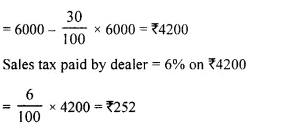Amount of the article inclusive of sales tax at which the dealer bought it
= ₹ 4200 + ₹ 252 = ₹ 4452
Dealer sells the article at a discount of 10% to the shopkeeper.
Price of the article which the shopkeeper paid to the dealer = ₹ 6000 – 10% of 6000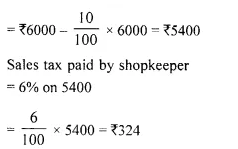Amount of the article inclusive of sales tax at which the shopkeeper bought it
= ₹ 5400 + ₹ 324 = ₹ 5724
The value added by dealer = ₹ 5400 – ₹ 4200 = ₹ 1200
Amount of VAT paid by dealer = 6% of 1200
= $$\frac { 6 }{ 100 }$$ x 1200 = ₹ 72
Price paid by shopkeeper including tax is ₹ 5724
VAT paid by dealer is ₹ 72

Hope given Selina Concise Mathematics Class 10 ICSE Solutions Chapter 1 Value Added Tax Ex 1C are helpful to complete your math homework.

If you have any doubts, please comment below. Learn Insta try to provide online math tutoring for you.